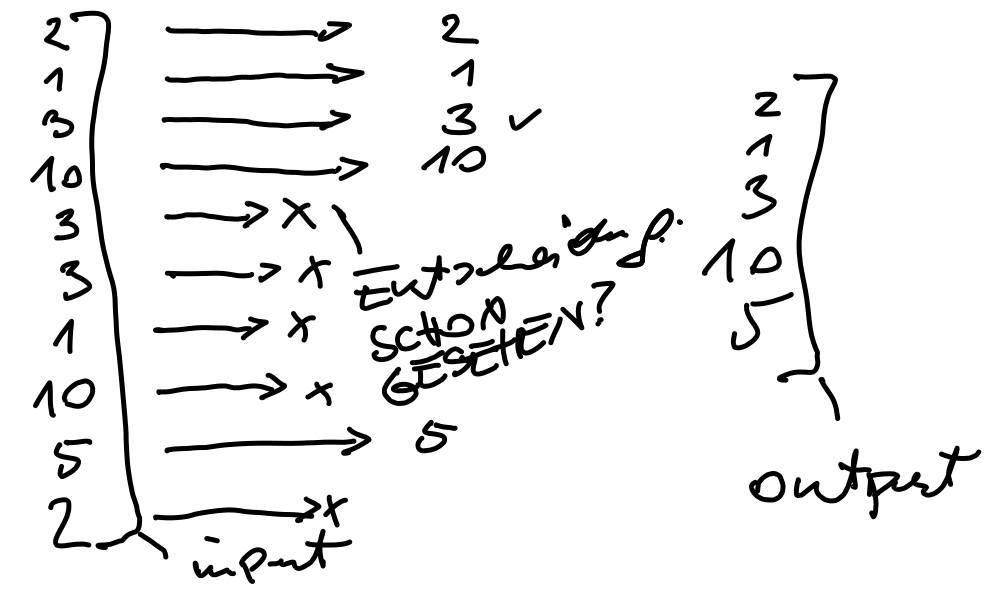# Python Basics (2021-11-17 - 2021-11-19)¶

## Day 2¶

• while Loops

• for Loops

• `while` and `for` in C (similar in Jave and C#): `for` is just a shorthand for `while` - as opposed to Python.

```#include <stdio.h>

int main(void)
{
int i = 0;
int s = 0;
while (i <= 10) {
s += i;
i += 1;
}
printf("sum (while): %d\n", s);

s = 0;
for (i = 0; i <= 10; i++)
s += i;
printf("sum (for): %d\n", s);

return 0;
}
```
• Sequential Datatypes

• Exercise: Function: uniq()```lin = [2,1,3,10,3,3,1,10,5,2]

lout = []
have = set()  # maintained in parallel to answer the "schon gesehen?"
# question faster

for element in lin:
if element not in have:
lout.append(element)

print('outputliste:', lout)
print('have:', have)
```
• File I/O

• Exercise: Parsing /etc/passwd

• Solution 1: relatively explicit and long

```import sys
import pprint

filename = sys.argv

userdatabase = {}

f = open(filename)
for line in f:
line = line.rstrip()   # remove linefeed at end of line
fields = line.split(':')

userid = int(fields)
groupid = int(fields)
description = fields
home = fields

userrecord = {}
userrecord['userid'] = userid
userrecord['groupid'] = groupid
userrecord['description'] = description
userrecord['home'] = home

# pprint.pprint(userrecord, indent=4)

#pprint.pprint(userdatabase, indent=4)

```
• Solution 2: dense, short, requires a bit of programming pratice (not much though)

```import sys
import pprint

filename = sys.argv

userdatabase = {}

f = open(filename)
for line in f:
'userid': userid,
'groupid': groupid,
'description': description,
'home': home,
}

```

## Day 3¶

• Back to Exercise: Function: uniq(): `set` usage for speed!

• The range Function

• Iteration, Comprehensions, and Generators

• Fibonacci Numbers: 1, 1, 2, 3, 5, 8, 13, …

```def fibo():
'''
Generate the *infinite* fibonacci sequence. Note that we use the
``yield`` statement and not ``return`` - this makes this a
*generator* which is fundamentally different from a *function*.
'''

first = second = 1

yield first
yield second

while True:
next = first + second
yield next
first, second = second, next

if __name__ == '__main__':    # started as program, and not imported
nums = fibo()
for i in nums:
print(i)
```
• Iterable and `dict`, `list`. See notebook.

• Comprehensions. See notebook.

• References, (Im)mutability

• Functions

• Modules and Packages

• CSV Files

The module `stock.py`:

```import csv
import datetime

# note: functions prefixed with '_' are not imported when someone says
# "from there import *". those functions are internal to this module,
# so it is good practice to hide them in such a way.

def _fix_float_number(record, fieldname):
old = record[fieldname]
old = old.replace(',', '.')
new = float(old)
record[fieldname] = new

def _fix_bool(record, fieldname):
old = record[fieldname]
if old == 'T':
new = True
elif old == 'F':
new = False
else:
new = True
record[fieldname] = new

def _fix_date(record, fieldname):
old = record[fieldname]
new = datetime.datetime.strptime(old, '%Y%m%d')
record[fieldname] = new

csvfile = open(filename)

converted_records = []
for record in rdr:
_fix_date(record, 'AS_OF_DATE')
_fix_bool(record, 'FUND_IN_UNIVERSE')
_fix_bool(record, 'FUND_OF_FUNDS')
_fix_float_number(record, 'FUND_HOLDINGS_COUNT')
_fix_float_number(record, 'FUND_HOLDING_FUNDS_COUNT')
converted_records.append(record)

return converted_records
```

The program `read-stock.py`:

```import stock
import sys
from pprint import pprint

pprint(record)
```
• Databases

• Exercise: Read CSV File, Write into Sqlite3 Database

The program `stock-to-db.py`:

```import stock

import sys
import sqlite3

csvname = sys.argv
dbname = sys.argv

db = sqlite3.connect(dbname)

stmt = f'''
insert into records values (
"{record['FUND_ISIN']}",
"{record['FUND_NAME']}",
"{record['FUND_TICKER']}"
);
'''
db.execute(stmt)

db.commit()
```

## PCAP Exam Syllabus¶

Exam block #1: Modules and Packages (12%)

Objectives covered by the block (6 items)

• import variants; advanced qualifiying for nested modules

Comment: `os.path`

• dir(); sys.path variable

• math: ceil(), floor(), trunc(), factorial(), hypot(), sqrt(); random: random(), seed(), choice(), sample()

• platform: platform(), machine(), processor(), system(), version(), python_implementation(), python_version_tuple()

• idea, __pycache__, __name__, public variables, __init__.py

• searching for modules/packages; nested packages vs directory tree

Exam block #2: Exceptions (14%)

Objectives covered by the block (5 items)

• except, except:-except; except:-else:, except (e1,e2)

• the hierarchy of exceptions

• raise, raise ex, assert

• event classes, except E as e, arg property

• self-defined exceptions, defining and using

Exam block #3: Strings (18%)

Objectives covered by the block (8 items)

• ASCII, UNICODE, UTF-8, codepoints, escape sequences

• ord(), chr(), literals

• indexing, slicing, immutability

• iterating through,

• concatenating, multiplying, comparing (against strings and numbers)

• in, not in

• .isxxx(), .join(), .split()

• .sort(), sorted(), .index(), .find(), .rfind()

Exam block #4: Object-Oriented Programming (34%)

Objectives covered by the block (12 items)

• ideas: class, object, property, method, encapsulation, inheritance, grammar vs class, superclass, subclass

• instance vs class variables: declaring, initializing

• __dict__ property (objects vs classes)

• private components (instance vs classes), name mangling

• methods: declaring, using, self parameter

• instrospection: hasattr() (objects vs classes), __name__, __module__, __bases__ properties

• inheritance: single, multiple, isinstance(), overriding, not is and is operators

• constructors: declaring and invoking

• polymorphism

• __name__, __module__, __bases__ properties, __str__() method

• multiple inheritance, diamonds

Exam block #5: Miscellaneous (List Comprehensions, Lambdas, Closures, and I/O Operations) (22%)

Objectives covered by the block (9 items)

• list comprehension: if operator, using list comprehensions

• lambdas: defining and using lambdas, self-defined functions taking lambda as as arguments; map(), filter();

• closures: meaning, defining, and using closures

• I/O Operations: I/O modes, predefined streams, handles; text/binary modes

• open(), errno and its values; close()